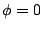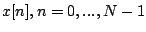Next: Wavetables and samplers Up: Sinusoids, amplitude and frequency Previous: More additive synthesis   Contents   Index

Exercises

1. A sinusoid (Page) has initial phaseand angular frequency. What is its period in samples? What is the phase at sample number?

2. Two sinusoids have periods of 20 and 30 samples, respectively. What is the period of the sum of the two?

3. If 0 dB corresponds to an amplitude of 1, how many dB corresponds to amplitudes of 1.5, 2, 3, and 5?

4. Two uncorrelated signals of RMS amplitude 3 and 4 are added; what's the RMS amplitude of the sum?

5. How many uncorrelated signals, all of equal amplitude, would you have to add to get a signal that is 9 dB greater in amplitude?

6. What is the angular frequency of middle C at 44100 samples per second?

7. Two sinusoids play at middle C (MIDI 60) and the neighboring C sharp (MIDI 61). What is the difference, in Hertz, between their frequencies?

8. How many cents is the interval between the seventh and the eighth harmonic of a periodic signal?

9. If an audio signalhas peak amplitude 1, what is the minimum possible RMS amplitude? What is the maximum possible?Next: Wavetables and samplers Up: Sinusoids, amplitude and frequency Previous: More additive synthesis   Contents   Index
Miller Puckette 2006-12-30